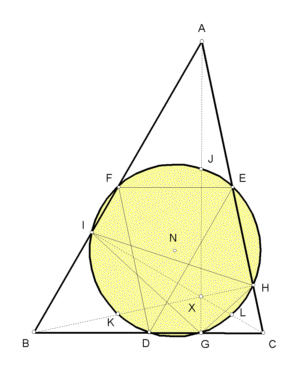# NINE-POINT CENTER

 In the plane of any triangle ABC, let D = the midpoint of side BC, E = the midpoint of side CA, F = the midpoint of side AB; G = foot on BC of altitude from A, H = foot on CA of altitude from B, I = foot on AB of altitude from C; J = midpoint of segment AX, K = midpoint of segment BX, L = midpoint of segment CX. (X, the orthocenter, is where the altitudes AG, BH, CI meet.) As you see in the sketch, a circle passes through all nine of the points D,E,F,G,H,I,J,K,L. It is the nine-point circle of triangle ABC, and its center, N, is the nine-point center.Triangle Centers